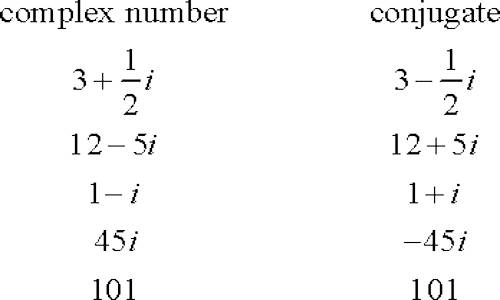Complex Numbers

The introduction of complex numbers plays a very important role in the theory of numbers.

A complex number is a number of the form a + bi, where ‘a’ and ‘b’ are real numbers and ‘I’ is an indeterminate satisfying i2 = −1. For example, 2 + 3i is a complex number.

The equations x2 + 5 = 0, x2 + 10 = 0, x2 = -1 are not solvable in the real number system i.e, these equations has no real roots. For example, i is the solution of the equation x2 = -1 and it has two solutions i.e., x = ± i, where √-1.

The number, ‘I’ is called an imaginary number. Generally, the square root of any negative real number is called imaginary number. The concept of imaginary numbers was first introduced by mathematician “Euler”. He was the one who introduced ‘i’ (read as ‘iota’) to represent √-1. He also defined i2 = -1.

Every number was positive after you squared it. So you couldn’t very well square-root a negative and expect to come up with anything sensible.

Definition of Complex number:

A complex number z is defined as an order pair of real numbers and is written as z = (a, b) or, z = a + ib, where a, b are real numbers and i = √-1.

In other words, in an ordered pair (a, b) of two real numbers a and b is represented by the symbol a + ib (where i = √-1) then the order pair (a, b) is called a complex number (or, an imaginary number).

Example of complex number:

3 + 2i, -1 + 5i, 7 – 2i, 2 + i√2, 1 + i, etc. are all complex numbers.Real and imaginary part of complex numbers:

According to the definition if the complex number (a, b) be denoted by z then z = (a, b) = a + ib (a, b ϵ R) where a is called the real part, denoted by Re(z) and b is called imaginary part, denoted by Im (z).

In other words, in z = a + ib (a, b ϵ R), if a = 0 and b = 1 then z = 0 + i ∙ 1 = i that is, i represents the unit of a complex quantity.

For this reason, the real number a is called the real part of the complex number z = a + ib and b is called its imaginary part.

In z = a + ib (a, b ϵ R), if b = 0 then z = (a, 0) = a + 0 ∙ i = a, (which is a real part) i.e., the complex number (a, 0) represents purely real number.

Again, in z = a + ib (a, b ϵ R), if a = 0 and b ≠ 0 then z = (0, b) = 0 + ib = ib which is called purely imaginary number

Therefore, a complex number z = a + ib (a, b ϵ R), reduces to a purely imaginary number when a = 0.

Equality of two complex numbers:

Two complex number z1 = a + ib and z2 = c + id

Two complex numbers z1 = (a, b) = a + ib and z2 = (c, d) = c + id are called equal, written as z1 = z2 if and only if a = c and b = d

In general, when real and imaginary parts of one of the complex number are respectively equal to the real and imaginary parts of the other complex number then they are equal.

For example, if the complex number z1 = x + iy and z2 = -8 + 3i are equal, then x = -8 and y = 3.

Complex numbers are useful abstract quantities that can be used in calculations and result in physically meaningful solutions. However, recognition of this fact is one that took a long time for mathematicians to accept.

Information Source;